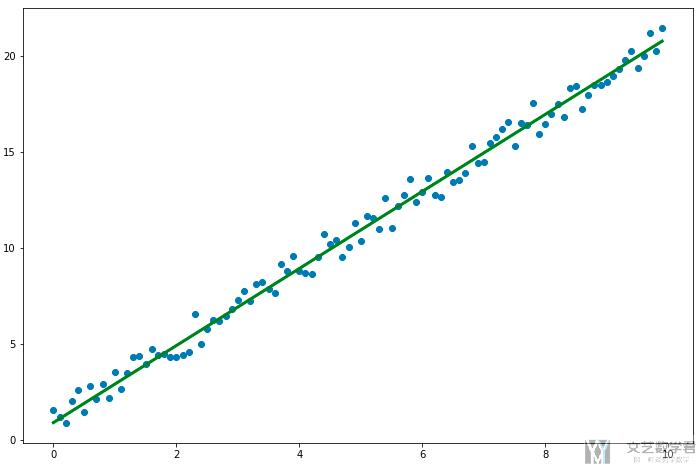# Pytorch入门教程07-Pytorch实现完整线性回归2020年10月6日07:55:20

2269字阅读7分33秒

Github仓库链接简单线性回归

## 完整的线性回归例子

### 模型的建立

1. class LinearRegression(nn.Module):
2.     def __init__(self):
3.         super(LinearRegression, self).__init__()
4.         self.linear1 = nn.Linear(1, 1)
5.     def forward(self, x):
6.         x = self.linear1(x)
7.         return x

• 自定的神经网络类必须继承 `nn.Module`.
• 自定义类中需要实现`__init__``forward`函数.
• `__init__`: 定义网络的结构.
• `forward`: 定义数据在模型中的传播路径.

1. # 模型初始化
2. linearModel = LinearRegression()

### 定义损失函数和优化器

1. loss = torch.nn.MSELoss() # 定义均方损失函数

1. # 定义一个SGD优化器
2. learning_rate = 0.001
3. optimizer = torch.optim.SGD(linearModel.parameters(), lr=learning_rate)

### 模型的训练

1. X_tensor = torch.from_numpy(X).view(100,1)
2. Y_tensor = torch.from_numpy(Y).view(100,1)

1. n_iters = 101
2. for epoch in range(n_iters):
3.     y_pred = linearModel(X_tensor)
4.     l = loss(Y_tensor, y_pred) # 求误差(注意这里的顺序)
5.     l.backward() # 求梯度
6.     optimizer.step()  # 更新权重，即向梯度方向走一步
8.     [w, b] = linearModel.parameters()  # 获得参数
9.     if epoch % 20 == 0:
10.         print(f'epoch {epoch+1}: w = {w.data}, loss = {l.item():.3f}')
11. print(f'根据训练模型预测, 当x=5时, y的值为： {linearModel(torch.tensor([5.0]))}')
12. """
13. epoch 1: w = tensor([[0.8991]]), loss = 49.043
14. epoch 21: w = tensor([[1.7334]]), loss = 3.314
15. epoch 41: w = tensor([[1.9409]]), loss = 0.481
16. epoch 61: w = tensor([[1.9924]]), loss = 0.305
17. epoch 81: w = tensor([[2.0050]]), loss = 0.294
18. epoch 101: w = tensor([[2.0080]]), loss = 0.294
20. """

#### 结果可视化

1. # 绘制预测曲线
2. y_pre = linearModel(X_tensor).detach().numpy()
3. fig = plt.figure(figsize=(12,8))
5. ax.scatter(X,Y)
6. ax.plot(X, y_pre, 'g-',  lw=3)
7. fig.show()## Pytorch训练步骤总结

1. 利用 `model(X)` 进行正向传播。
2. 利用 `loss(Y, y_predicted)` 计算模型损失。
3. 利用 `loss.backward()` 计算模型梯度。
4. 利用 `optimizer.step()` 更新权重。
5. 利用 `optimizer.zero_grad()` 清空梯度。
6. 重复 1-5 的操作。

• 微信公众号
• 关注微信公众号
•• QQ群
• 我们的QQ群号
•• 本文由 发表于 2020年10月6日07:55:20
• 转载请务必保留本文链接：https://mathpretty.com/12516.html